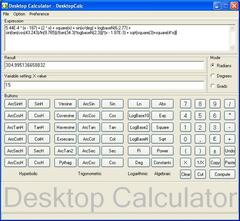Search
Software
Options for Desktop Calculator - DesktopCalc
Desktop Calculator - DesktopCalcDesktopCalc is an enhanced, easy-to-use and powerful scientific calculator with an expression editor, printing operation, result history list and integrated help. Desktop calculator gives students, teachers, scientists and engineers the power to find values for even the most complex equation set.

DesktopCalc uses Advanced DAL (Dynamic Algebraic Logic) mechanism to perform all its operation with the built-in 38-digit precision math emulator for high precision.

DesktopCalc combines fast "just-one-click" interface with broad set of functions. It was designed as a tool that is convenient for both elementary and scientific calculator. DesktopCalc features include the following:
* Possibility to enter mathematical formulas as with a keyboard as with built-in button-panels.
* Scientific calculations - unlimited expression length
* Syntax highlight of math expression and proper operator precedence
* Parenthesis compatible
* Scientific notation
* Calculation range:
- maximal positive number: 1.797E+308
- minimal positive number: 2.225E-308
* All functions, mathematical and physical constants can also be used in expression
- 40 trigonometric, logarithmic, hyperbolic and algebraic functions
- 50 built-in most common mathematical and physical constants
* Clipboard smart functionality
- Cut, Copy and Paste operations